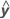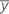# Methods and formulas for the analysis of variance in One-Way ANOVA

Select the method or formula of your choice.

## Degrees of freedom (DF)

### Formula

Indicates the number of independent elements in the sum of squares. The degrees of freedom for each component of the model are:
• DF (Factor) = r – 1
• DF Error = nTr
• Total = nT – 1

### Notation

TermDescription
nT total number of observations
r number of factor levels

## Sum of Squares (SS)

### Formula

The sum of squared distances. SS Total is the total variation in the data. SS (Factor) is the deviation of the estimated factor level mean around the overall mean. It is also known as the sum of squares between treatments. SS Error is the deviation of an observation from its corresponding factor level mean. It is also known as error within treatments.

The calculations are:

### Notation

TermDescription
i . mean of the observations at the i th factor level
y̅.. mean of all observations
yij value of the j th observation at the i th factor level

## Mean squares (MS)

### Formula

The calculation for the mean square for the factor follows:

The calculation for the mean square for error follows:

### Notation

TermDescription
MSMean Square
SSSum of Squares
DFDegrees of Freedom

## F-value

### Formula

The degrees of freedom for the numerator are r – 1. The degrees of freedom for the denominator are nTr.

### Notation

TermDescription
nT total number of observations
r number of factor levels

## P-value

Used in hypothesis tests to help you decide whether to reject or fail to reject a null hypothesis. The p-value is the probability of obtaining a test statistic that is at least as extreme as the actual calculated value, if the null hypothesis is true. A commonly used cut-off value for the p-value is 0.05. For example, if the calculated p-value of a test statistic is less than 0.05, you reject the null hypothesis.

## S

An estimate of σ, the measure of the within-sample standard deviation. Note that S2 = MS Error. This is equivalent to the pooled standard deviation used in calculating the individual confidence intervals.

## R-sq

Another presentation of the formula is:

R2 can also be calculated as the squared correlation of y and.

### Notation

TermDescription
SSSum of Squares
yresponse variablefitted response variable

### Notation

TermDescription
MSMean Square
SSSum of Squares
DFDegrees of Freedom

## R-sq (pred)

While the calculations for R2(pred) can produce negative values, Minitab displays zero for these cases.

### Notation

TermDescription
yi i th observed response valuemean response
n number of observations
ei i th residual
hi i th diagonal element of X(X'X)–1X'
X design matrix
By using this site you agree to the use of cookies for analytics and personalized content.  Read our policy